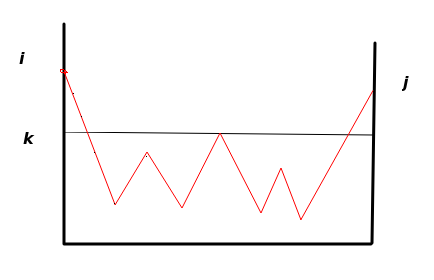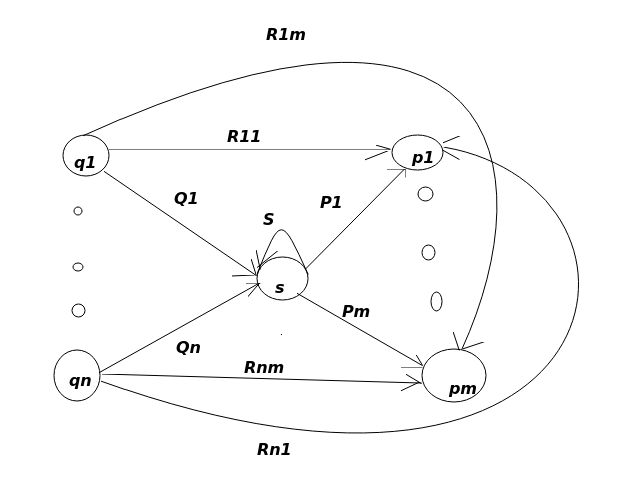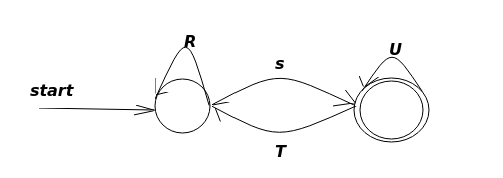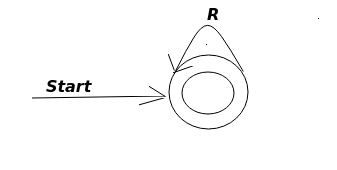# 自动机：DAF,NAF,正则表达式的关系

## DAF 转化为正则表达式

### 分解问题

• 什么叫最高只经过状态$k$,$k$属于$\{1,2,3...n\}$? 这里指的是从状态i到状态j路径中的状态最高不超过k。
• 状态$i$,$j$,$k$直接有什么相互的联系吗? 这里并没有必然的联系，$i$可以等于$j$，而且状态$i$$j$可以大于$k$，也可以小于$k$垂直方向为状态，这里我们取每个顶点为某个具体的状态，线段表示状态的转移，可以看到这里虽然有2次经过了状态$k$，但是都是两个端点，除两个端点状态之外，并没有经过大于状态$k$的，状态转移。这幅图在这里表示为带有属于正则表达式$R_{ij}^{(k)}$的语言的标记的路径。

### 通过消除法将自动机转换为正则表达式• 对于每个接受状态$q$,从$q_0$开始，消除所有中间状态，产生一个等价的自动机，所有箭弧用正则表达式来表示。最后只留下接受状态$q$$q_0$,再把所有$q_0$到接受状态$q$的正则表达式取并。
• 如果$q\neq q_0$,最后状态图如下,其路径可以用正则表达式$(R+SU^*T)^*SU^*$,其中$(R+SU^*T)^*$表示$q_0$自身环和经过$q$又回到$q_0$的环：• 如果初始状态和接受状态相同，那就相当于去掉了所有状态，只留下了初始状态，只剩下一个单状态的自动机